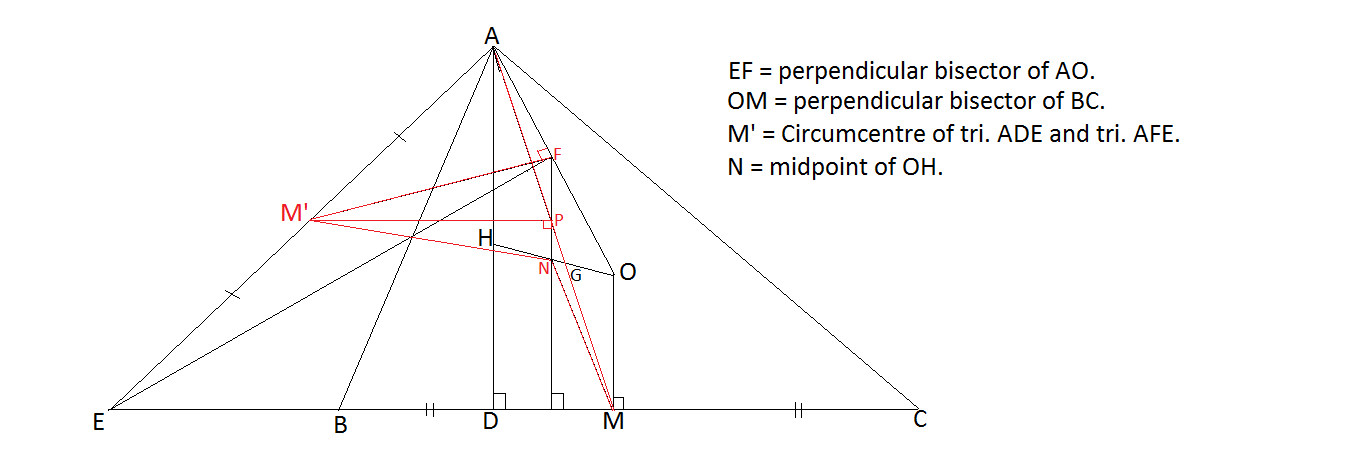# Xuming's Synthetic Geometry Group - Sharky's Submissions

My problems are from the Australian School of Excellence I went to last year from the worksheets.

1. Acute triangle $$ABC$$ has circumcircle $$\Gamma$$. The tangent at $$A$$ to $$\Gamma$$ intersects $$BC$$ at $$P$$. Let $$M$$ be the midpoint of the segment $$AP$$. Let $$R$$ be the second intersection point of $$BM$$ with $$\Gamma$$. Let $$S$$ be the second intersection point of $$PR$$ with $$\Gamma$$. Prove $$CS$$ is parallel to $$PA$$.

2. Let $O$ be the circumcentre of acute $\Delta ABC$, $H$ be the orthocentre. Let $AD$ be the altitude of $\Delta ABC$ from $A$, and let the perpendicular bisector of $AO$ intersect $BC$ at $E$. Prove that the circumcircle of $\Delta ADE$ passes through the midpoint of $OH$.Note by Sharky Kesa
5 years, 10 months ago

This discussion board is a place to discuss our Daily Challenges and the math and science related to those challenges. Explanations are more than just a solution — they should explain the steps and thinking strategies that you used to obtain the solution. Comments should further the discussion of math and science.

When posting on Brilliant:

• Use the emojis to react to an explanation, whether you're congratulating a job well done , or just really confused .
• Ask specific questions about the challenge or the steps in somebody's explanation. Well-posed questions can add a lot to the discussion, but posting "I don't understand!" doesn't help anyone.
• Try to contribute something new to the discussion, whether it is an extension, generalization or other idea related to the challenge.

MarkdownAppears as
*italics* or _italics_ italics
**bold** or __bold__ bold
- bulleted- list
• bulleted
• list
1. numbered2. list
1. numbered
2. list
Note: you must add a full line of space before and after lists for them to show up correctly
paragraph 1paragraph 2

paragraph 1

paragraph 2

[example link](https://brilliant.org)example link
> This is a quote
This is a quote
    # I indented these lines
# 4 spaces, and now they show
# up as a code block.

print "hello world"
# I indented these lines
# 4 spaces, and now they show
# up as a code block.

print "hello world"
MathAppears as
Remember to wrap math in $$ ... $$ or $ ... $ to ensure proper formatting.
2 \times 3 $2 \times 3$
2^{34} $2^{34}$
a_{i-1} $a_{i-1}$
\frac{2}{3} $\frac{2}{3}$
\sqrt{2} $\sqrt{2}$
\sum_{i=1}^3 $\sum_{i=1}^3$
\sin \theta $\sin \theta$
\boxed{123} $\boxed{123}$

Sort by:

Question 2. Let's call the midpoint of $AO$ and $OH$, $P$ and $N$ respectively. Since $\angle APE = \angle ADE$, this implies that quadrilateral $APDE$ is cyclic. Therefore it suffices to prove that quadrilateral $APND$ is cyclic as well.

Since $\frac{OP}{AO} = \frac{ON}{OH}$, we know that $PN || AD$.

We also know that $N$ is the center of the nine-point circle, and $D$ is on the circle, therefore $ND = \frac{R}{2}$. We also know that $PA = \frac{AO}{2} = \frac{R}{2}$. This implies that $APND$ is a isosceles trapezoid which implies that this is cyclic and we are done.

- 5 years, 10 months ago

Nicely done.

- 5 years, 10 months ago

@Nihar Mahajan ,@Mehul Arora , @Surya Prakash , @Agnishom Chattopadhyay , @Alan Yan , @Shivam Jadhav , @Swapnil Das , @Mardokay Mosazghi , @Kushagra Sahni , @Xuming Liang Post your solutions please. Sorry for the late submission.

- 5 years, 10 months ago

So , finally you posted.I was waiting for you shraky :P

- 5 years, 10 months ago

I had no internet for the last 2 days. I was transitioning to a more permanent wi-fi connection.

- 5 years, 10 months ago

Hints: 1. power of a point/radical axis configuration/similarity 2. reflect a certain point.

- 5 years, 10 months ago

Here is an another one for the second without using Nine Point Circles.

- 4 years, 4 months ago

@Sharky Kesa Is it from Australian School of Excellence?

- 4 years, 4 months ago

Yes, this was from the Junior problems in the School of Excellence from 2 years ago.

- 4 years, 4 months ago- 4 years, 4 months ago

Solution: 2 :: (\Since F lies on Circumcircle of ADE. It is sufficient enough to prove that M'F = M'N.

OM' || FN. Also, OM = 1/2 AH (Euler rule) And FN = 1/2 AH (Midpoint theorem) => OM = FN.

=> MNFO is a ||gm. => NM || AF and NM = FO = AF

'.' NM || AF => ∠ AFP = ∠ MNP , ∠ APF = ∠ MPN and ON = AF. => Tri. AFP = Tri. MNP.

=> AP = PM and FP = NP. ---- (1)

Also AP = PM => M'P || EC => M'P perp. FN ---- (2)

The two results (1) and (2) tells us that M'F = M'N/)

Sorry for the inconvenience of Latex. I have just learnt to write italics only.

- 4 years, 4 months ago

Question 1:

From Power of a Point,

$MB \times MR = MA^2 = MB^2$

Thus, $\triangle MPB \sim \triangle MRP$. Thus, $\angle APC = \angle PRB = \angle SCP$

where the last follows from SCQR cyclic.

Thus we can conclude.

- 4 years, 4 months ago

BRSC cyclic I mean

- 4 years, 4 months ago